# How to write C++ Program

## How to write C++ Program?

In this article, I am going to discuss how to write the basic C++ Program. Please read our previous article where we discussed the Basic Structure of the C++ Program. At the end of this article, you will understand the steps required to write a basic C++ Program.

##### Steps for writing programs in C++

Let us learn the steps for writing basic programs in C++. Every program will have three elements.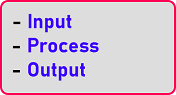Take Input, process them and give the output. Suppose, we have a program that adds two numbers. Then we should know two numbers. First of all, we will input two numbers. Then we will add them and we will give the output.

Now let us take another example. Suppose we have a program for finding the area of a rectangle. So, we should know the length and breadth of the rectangle, and then we need to multiply them and give the area result as output. Now let us see how we can follow these steps for writing a simple program for adding two numbers using C++ Language.

##### Flowchart for adding two numbers

First of all, we will draw a flowchart for adding two numbers, and then we will take our algorithm using pseudocode. Then will write down a C++ program for adding two numbers. First, let us draw a flow chart. So, what are the things we have to do? Let us draw a starting point of a flow chart that is start.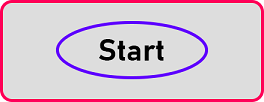Then our program should take 2 numbers as input. For input and output values we use parallelogram. We will draw a parallelogram and print a message for input as: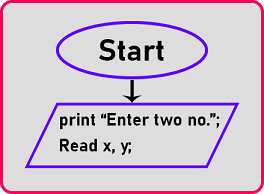Here we have printed “Enter two no.” and after that, we have to read two numbers which are x and y and these are called variables. Now we have two numbers, we have to add them. For the process, the rectangle shape is used. So the next step is process: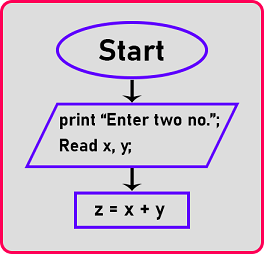Here we have added ‘x’ and ‘y’ and store the result in the ‘z’ variable. Now we have the result ready, we should give the result to the user that some of the two numbers is, so for output again draw the parallelogram, and give the output here.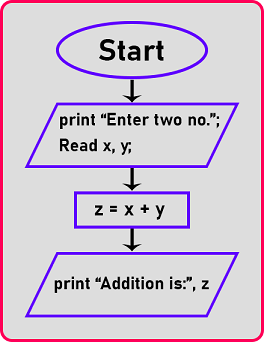So here we will see ‘Addition is:’ after it, the value of ‘z’ will be printed on the screen. We have performed the addition of two numbers. Now we have to end this flow chart and for that, we will draw an oval: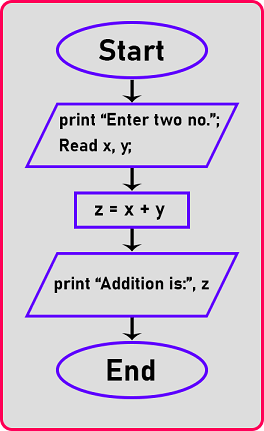This will stop here. Oval or ellipses is the shape that we use to start or end the flow chart. This is how we can draw the flow chart for adding two numbers.

##### Algorithm or Pseudocode for Adding two numbers:

Now the same thing we will write as an algorithm using pseudocode. Let us write an algorithm for adding two numbers: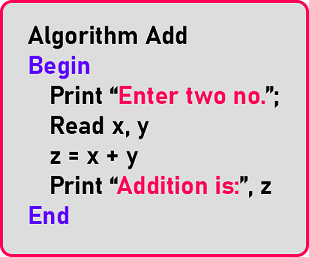This is the algorithm for adding two numbers. Here we write the same thing as pseudocode which we explained in the flow chart. So, these are the steps we have completed and written them as an algorithm.

##### C++ Program for adding two numbers

Now, we have to write a C++ program for adding two numbers. So let us write the C++ code. The following is the complete code for adding 2 numbers in C++.

```#include <iostream>
using namespace std;
int main()
{
int x, y, z;
cout << "Enter 2 numbers: ";
cin >> x >> y;
z = x + y;
cout << "Addition is " << z;
return 0;
}
```
###### Output: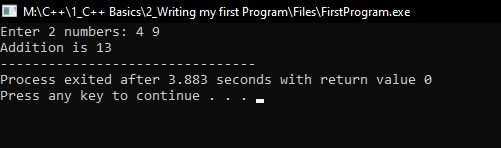Let’s see how we write the code: First, we have written the library file ‘iostream’. Next, we have written the namespace so that we can use the object which is present in ‘iostream’ i.e. ‘cout’, ‘cin’. Next, we write down the main function with parenthesis and then open and curly braces.

Now the main code which we have explained in the process part in the flowchart has to be written inside the main function. Then we print a message on the screen to ask the user for input and then we performed addition on the input and print the result on the screen. And so here inside the main function, we must write down return 0. So that’s all.

If we miss anything then the compiler will not compile our program, so we should write the things that are understandable by the compiler. So, we have written everything properly. This is a program in C++ for adding two numbers.

##### Another Program in C++:

Now we will continue and show you how to read a name in C++ and give a message. The name means a string or set of characters. It is a simple program. Now let us write a program in which we will take the name of a user and display a welcome message with the name.

```#include <iostream>
using namespace std;
int main ()
{
string name;
cout << "Enter your name: ";
getline (cin, name);
cout << "Welcome " << name;
return 0;
}
```
###### Output: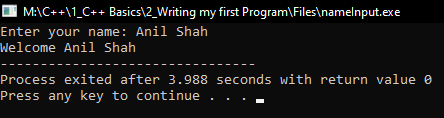Now one thing we have to learn is that in the above program, we haven’t used only cin but we use getline (cin, name). The getline is a standard library function that is used to read a string or a line from an input stream.

If we use cin only then it will not read the complete name i.e. Rahul Singh, it will only store Rahul. So, for storing the full name we have to use getline, it read the whole line until we press enter.

##### FAQs
###### Why return 0;

What is the meaning or use of return 0; here and what will happen if we don’t use return 0 in our code. When a program is ending it should return 0. It is like a standard in C++ programs, it must be written. return 0; means the program has terminated successfully. In some compiler programs may run without return 0; also.

###### How to read multiple words? or how to read multiple words in a name?

If you want to read more than one word, then use getline. Include a header file #include<string> or #include<cstring>
getline(cin,name);

###### Difference between int main() and void main()

In C++ int main() is standard. Some compiler allow void main() also.

###### It is mandatory to write return 0;?

It is be9er to write return 0; Some compilers may compile the program without return 0; also.

###### What is cin.ignore()?

Unable to read a string after reading a number. I am not able to get input using getline if do some before it. Is there any reason for that?
If your program looks like this

```int main()
{
int x;
string str;
cout<<"Enter number";
cin>>x; // When you enter a number and hit enter
cint>>str; // This str will take that enter key and will not read a string.
}
```

After entering a number from the keyboard we hit the enter key. That enter key remains in the input buffer and cin>>str; will consider it as input and stops. We should clear the input buffer before reading a string. The cin.ignore() is used for clearing the buffer.

###### What is this endl?

The endl is used for giving a new line in the output. Just like \n
cout<<“hi”<<“bye”; will print like this hibye
cout<<“hi”<<endl<<“bye”; will print on the screen like this
hi
bye
Note: bye will print in the next line.

###### What are the Differences between variables and Objects?

Variables are names given to values. variable of a class is called an object. like
int x=10;
x is of type primitive data type (int), it is a variable.
string name=”Smith”;
name is a variable of type string. The string is a class in C++. So, name is an object.

In the next article, I am going to discuss Why we need Data Types in C++ with Examples. Here, in this article, I try to explain how to write a basic C++ program and I hope you enjoy this how to write a basic C++ Program article.